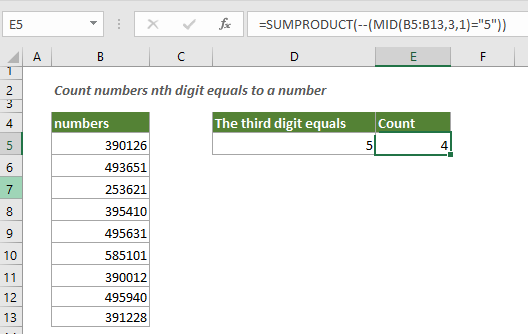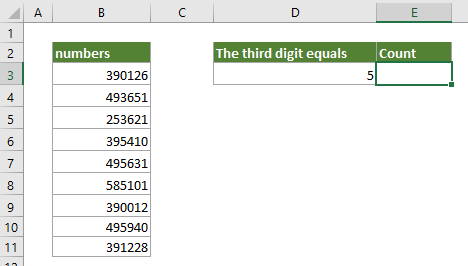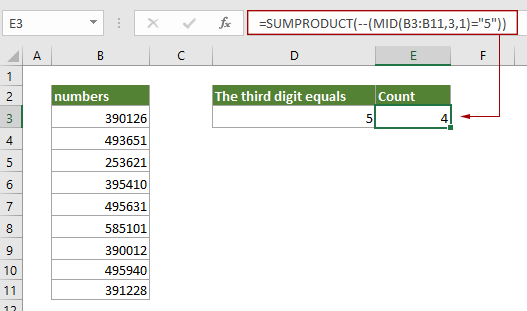## Count numbers where the nth digit equals to a given number

This tutorial provides a formula based on the SUMPRODUCT and the MID function to count numbers where the nth digit equals to a given number in Excel.#### How to count numbers where the nth digit equals to a given number?

Supposing there is a number list in range B3:B11, to count the number of cells which the third digit equals to 5, you can apply the below formula.Generic Formula

=SUMPRODUCT(--(MID(range,digit,n)="number"))

Arguments

Range: The range of cells lists all sheet names of current workbook.
Digit: The range of cells you want to count the specific value from.
Number: The particular text you will count across sheets.
N:The length of number value.

How to use this formula?

1. Select a blank cell to output the result.

2. Enter the below formula into it and press the Enter key to get the result.

=SUMPRODUCT(--(MID(B3:B11,3,1)="5"))Note: In this formula, B3:B11 is the column range contains the numbers you want to count, number 3 and 5 indicate you will check if the third digit of numbers equals to 5, you can change them based on your need.

How this formula works?

=SUMPRODUCT(--(MID(B3:B11,3,1)="5"))

• MID(B3:B11,3,1): The MID function finds the third digit from the middle of numbers in range B3:B11 and returns an array like this:{"0";"3";"3";"5";"5";"5";"0";"5";"1"}.
• {"0";"3";"3";"5";"5";"5";"0";"5";"1"}="5": Here compare each number in the array with “5”, if match, returns TRUE, otherwise, returns FALSE. You will get an array as {FALSE;FALSE;FALSE;TRUE;TRUE;TRUE;FALSE;TRUE;FALSE}.
• --({FALSE;FALSE;FALSE;TRUE;TRUE;TRUE;FALSE;TRUE;FALSE}): These two minus signs convert “TRUE” into 1 and “FALSE” into 0. Here you will get a new array as {0;0;0;1;1;1;0;1;0}.
• =SUMPRODUCT{0;0;0;1;1;1;0;1;0}:The SUMPRODUCT function sums all numbers in the array and returns the final result as 4.

#### Related functions

Excel SUMPRODUCT function
The Excel SUMPRODUCT function can be used to multiply two or more columns or arrays together, and then get the sum of products.

Excel MID function
The Excel MID function is used to find and return a specific number of characters from the middle of given text string.

#### Related formulas

Count numbers that begin with a specific number
This tutorial provides a formula based on the SUMPRODUCT and LEFT functions to count number of cells containing numbers that begin with a specific number(s) in Excel.

Count multiple criteria with NOT logic in Excel

Count or sum only whole numbers in Excel
This post provides two formulas based on the SUMPRODUCT function and the MOD function to help you count and sum only whole numbers in a range of cells in Excel.

Count Occurrences Of Particular Text In Entire Excel Workbook

### The Best Office Productivity Tools

#### Kutools for Excel - Helps You To Stand Out From Crowd

Would you like to complete your daily work quickly and perfectly? Kutools for Excel brings 300 powerful advanced features (Combine workbooks, sum by color, split cell contents, convert date, and so on...) and save 80% time for you.

• Designed for 1500 work scenarios, helps you solve 80% Excel problems.
• Reduce thousands of keyboard and mouse clicks every day, relieve your tired eyes and hands.
• Become an Excel expert in 3 minutes. No longer need to remember any painful formulas and VBA codes.
• 30-day unlimited free trial. 60-day money back guarantee. Free upgrade and support for 2 years.#### Office Tab - Enable Tabbed Reading and Editing in Microsoft Office (include Excel)

• One second to switch between dozens of open documents!
• Reduce hundreds of mouse clicks for you every day, say goodbye to mouse hand.
• Increases your productivity by 50% when viewing and editing multiple documents.
• Brings Efficient Tabs to Office (include Excel), Just Like Chrome, Firefox, And New Internet Explorer.